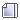###Author Topic: geographial isolations role in evolution  (Read 2507 times)

####Numsgil##### geographial isolations role in evolution
« Reply #15 on: October 11, 2005, 06:09:15 PM »
Quote
added:
Now I have got an idea a repulsive,  force that first get greater with distanc ebefore becoming weaker.
for example.

G*m1*m2 *(r/20)^2/(r/20)^3
That's an interesting idea.  Maybe some sort of way to describe forces in terms of an equation.  Custom forces could be really neat.

A computational note: r^3 will slow the program down dramatically.  r^2 is easy to calculate sicne you don't take a sqr root.  That is, you can just do r^2 = a^2 + b^2 (pythagorous theorem).

r^3 on the other hand is sort of a mess.

Negative G values work jsut as well, but I'm not sure they'd be terribly interesting or not.  I mean, eventually you'd get a kind of uniform distriubtion.